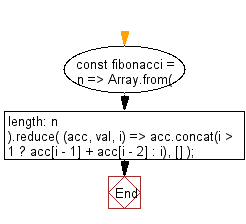# JavaScript: Generate an array, containing the Fibonacci sequence, up until the nth term

## JavaScript fundamental (ES6 Syntax): Exercise-237 with Solution

Write a JavaScript program to generate an array containing the Fibonacci sequence, up to the nth term.

• Use Array.from() to create an empty array of the specific length, initializing the first two values (0 and 1).
• Use Array.prototype.reduce() and Array.prototype.concat() to add values into the array, using the sum of the last two values, except for the first two.

Sample Solution:

JavaScript Code:

``````//#Source https://bit.ly/2neWfJ2
const fibonacci = n =>
Array.from({ length: n }).reduce(
(acc, val, i) => acc.concat(i > 1 ? acc[i - 1] + acc[i - 2] : i),
[]
);
console.log(fibonacci(2));
console.log(fibonacci(3));
console.log(fibonacci(6));
```
```

Sample Output:

```[0,1]
[0,1,1]
[0,1,1,2,3,5]
```

Flowchart:Live Demo:

See the Pen javascript-basic-exercise-237-1 by w3resource (@w3resource) on CodePen.

Improve this sample solution and post your code through Disqus

What is the difficulty level of this exercise?

Test your Programming skills with w3resource's quiz.

﻿